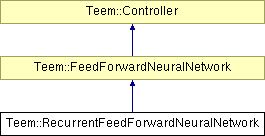# Teem::RecurrentFeedForwardNeuralNetwork Class Reference [Controllers and neural networks]

`#include <FeedForwardNeuralNetwork.h>`

Inheritance diagram for Teem::RecurrentFeedForwardNeuralNetwork:List of all members.

## Detailed Description

Feed forward neural network with optional recurence for each layer.

## Public Member Functions

RecurrentFeedForwardNeuralNetwork (size_t inputCount, size_t outputCount, const std::string &root)
Constructor, create a recurrent neural network with inputCount inputs and outputCount outputs.
virtual ~RecurrentFeedForwardNeuralNetwork ()
Destructor.
virtual void step ()
Propagate the input values to the output through all the layers.
virtual void randomize (double from, double to)
Put random weights with uniform distribution.
void setRecursiveWeight (size_t layer, size_t from, size_t to, double w)
Set the recursive weight in a layer.
double getRecursiveWeight (size_t layer, size_t from, size_t to) const
Get the value for a particular recursive weight.
bool isLayerRecursive (size_t layer)
Return true if layer is recurrent.

## Protected Attributes

Ishtar::Variable< bool > outputLayerRecursive
is output layer recursive?
std::vector< Matrix< double > > recursiveWeights
recusive weights for each layer
std::vector< bool > layerRecursive
is layer recursive?

The documentation for this class was generated from the following files:

Generated on Mon Oct 24 17:38:27 2005 for Teem by1.4.2